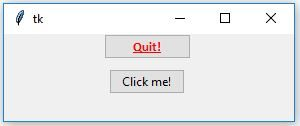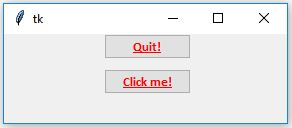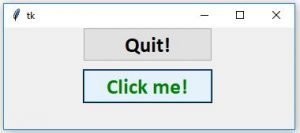# Python | Add style to tkinter button

Tkinter is a Python standard library which is used to create GUI (Graphical User Interface) applications. It is one of the most commonly used package of Python. Tkinter supports both traditional and modern graphics support with the help of `Tk` themed widgets. All the widgets that tkinter has also available in tkinter.ttk.

Adding style in a `tkinter.ttk` button is little bit creepy because it doesn’t support direct implementation. To add styling in a `ttk.Button` we have to first create a object of style class which is available in `tkinter.ttk`.

We can create ttk.Button by using following steps:

`btn = ttk.Button(master, option = value, ...)`

ttk.Button options –

command: A function to be called when button is pressed.
text: Text which appears on the Button.
image: Image to be apper on the Button.
style: Style to be used in rendering this button.

To add styling on the `ttk.Button` we cannot directly pass the value in the options. Firstly, we have to create a Style object which can be created as follows:

`style = ttk.Style()`

Below code will be adding style to only selected Buttons i.e, only those buttons will get changed in which we will be passing style option.

Code #1:

 `from` `tkinter ``import` `*`  `from` `tkinter.ttk ``import` `*`  ` `  `root ``=` `Tk() ` `root.geometry(``'100x100'``) ` ` `  `# This will create style object ` `style ``=` `Style() ` ` `  `# This will be adding style, and  ` `# naming that style variable as  ` `# W.Tbutton (TButton is used for ttk.Button). ` ` `  `style.configure(``'W.TButton'``, font ``=` `               ``(``'calibri'``, ``10``, ``'bold'``, ``'underline'``), ` `                ``foreground ``=` `'red'``) ` ` `  `# Style will be reflected only on  ` `# this button because we are providing ` `# style only on this Button. ` `''' Button 1'''` `btn1 ``=` `Button(root, text ``=` `'Quit !'``,  ` `                ``style ``=` `'W.TButton'``, ` `             ``command ``=` `root.destroy) ` `btn1.grid(row ``=` `0``, column ``=` `3``, padx ``=` `100``) ` ` `  `''' Button 2'''` `btn2 ``=` `Button(root, text ``=` `'Click me !'``, command ``=` `None``) ` `btn2.grid(row ``=` `1``, column ``=` `3``, pady ``=` `10``, padx ``=` `100``) ` ` `  `root.mainloop() `

Output:Only one button will get styled because in the above code we are providing styling only in one Button.

Code #2 Apply style on all the available buttons

 `from` `tkinter ``import` `*`  `from` `tkinter.ttk ``import` `*`  ` `  `root ``=` `Tk() ` `root.geometry(``'100x100'``) ` ` `  `style ``=` `Style() ` ` `  ` `  `# Will add style to every available button  ` `# even though we are not passing style ` `# to every button widget. ` `style.configure(``'TButton'``, font ``=`  `               ``(``'calibri'``, ``10``, ``'bold'``, ``'underline'``), ` `                ``foreground ``=` `'red'``) ` `# button 1 ` `btn1 ``=` `Button(root, text ``=` `'Quit !'``,  ` `                  ``style ``=` `'TButton'``, ` `             ``command ``=` `root.destroy) ` ` `  `btn1.grid(row ``=` `0``, column ``=` `3``, padx ``=` `100``) ` ` `  `# button 2 ` `btn2 ``=` `Button(root, text ``=` `'Click me !'``, command ``=` `None``) ` `btn2.grid(row ``=` `1``, column ``=` `3``, pady ``=` `10``, padx ``=` `100``) ` ` `  `root.mainloop() `

Output:Now if you want to change the appearance of the buttons by the movement of mouse i.e, now when we hover mouse over the button it will change it’s color when we press it will change color and so on.

Code #3 Change color on mouse hover

 `from` `tkinter ``import` `*` `from` `tkinter.ttk ``import` `*`  ` `  `root ``=` `Tk() ` `root.geometry(``'500x500'``) ` ` `  `style ``=` `Style() ` `style.configure(``'TButton'``, font ``=`  `               ``(``'calibri'``, ``20``, ``'bold'``), ` `                    ``borderwidth ``=` `'4'``) ` ` `  `# Changes will be reflected ` `# by the movement of mouse. ` `style.``map``(``'TButton'``, foreground ``=` `[(``'active'``, ``'! disabled'``, ``'green'``)], ` `                     ``background ``=` `[(``'active'``, ``'black'``)]) ` ` `  `# button 1 ` `btn1 ``=` `Button(root, text ``=` `'Quit !'``, command ``=` `root.destroy) ` `btn1.grid(row ``=` `0``, column ``=` `3``, padx ``=` `100``) ` ` `  `# button 2 ` `btn2 ``=` `Button(root, text ``=` `'Click me !'``, command ``=` `None``) ` `btn2.grid(row ``=` `1``, column ``=` `3``, pady ``=` `10``, padx ``=` `100``) ` ` `  `root.mainloop() `

Output:My Personal Notes arrow_drop_upCheck out this Author's contributed articles.

If you like GeeksforGeeks and would like to contribute, you can also write an article using contribute.geeksforgeeks.org or mail your article to contribute@geeksforgeeks.org. See your article appearing on the GeeksforGeeks main page and help other Geeks.

Please Improve this article if you find anything incorrect by clicking on the "Improve Article" button below.

Article Tags :

1

Please write to us at contribute@geeksforgeeks.org to report any issue with the above content.